# MOSFET (Si/SiC) Forum Discussions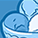Level 1Level 1

# PLECS model vs SPICE model

I have been simulating the Infineon IMW120R030M1H in LTSpice and PLECS (using the PLECS blockset with Simulink). Both models are provided on this website.

I have set up an identical double pulse test in both softwares and tested the Infineon device. I am aware that PLECs models switching devices ideally and then uses look up tables for losses. Am I right to say that as Infineon have provided both models they will behave the same under the same conditions? I have tested this theory and the PLECs model has higher losses but lower temperature rise.

I understand if the temperature is not identical as the softwares model thermal environments differently-  but surely the losses should be very similar?

Cumulative losses: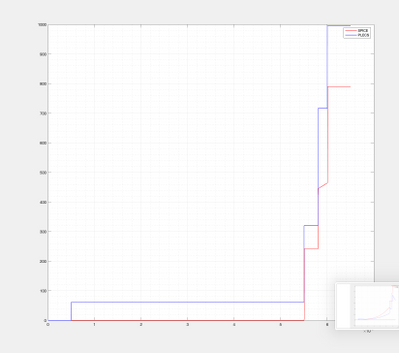Temperature: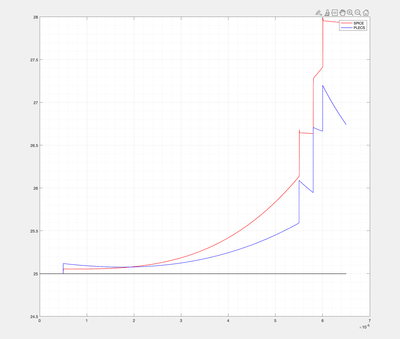Vgs to Device: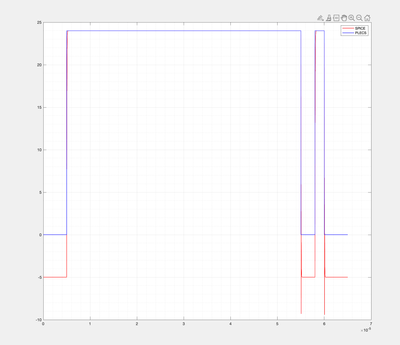Switching Losses: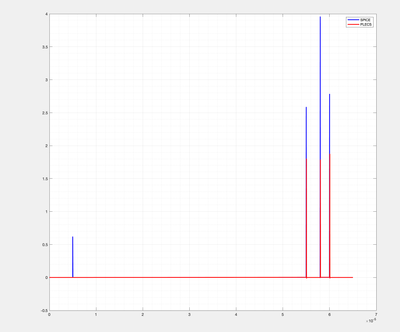Schematics: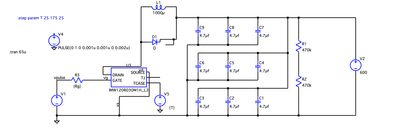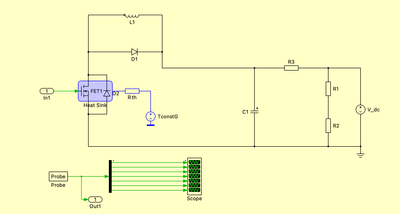Thankyou in advance, let me know if any questions!

1 SolutionModeratorModerator

# Re: PLECS model vs SPICE model

Hello Hugoc,

We can conclude that the output of the PLECS simulation is power in Watts, and not energy in Joules.
I am glad you could get energy levels close to the SPICE simulation.

I understand that there is still a difference in the resulting energies.
As with time the error of the energy calculations will get bigger and bigger, I suggest you compare the instantaneous power instead.

Let me know about these three points:
1) Where did you obtain the simulation model for SPICE? On our product page, the simulation files provided are for the IMBGxxxxx series.
I assume you are using the IMBGxxxxx model. This is almost identical to the IMW part, but there are still differences in the switching losses.

2) When simulating and integrating the power from the PLECS output, what step size are you using? This can make a big impact on the resulting calculated energy.
Ideally, we would like to have a short a step as possible. Again, this problem will be lessened if just using power is the variable to compare.

3) Your SPICE simulation waveforms are very ideal. When simulating in SPICE on my side, there are some natural fluctuations due to the non-ideal nature of the components.
For example, the first turn-on should have some current peak.

4) This is more of a comment, but I went over the .xml file information.
Both turn-on and turn-off have a minimum energy loss assigned.
Therefore, even if the current through the device is 0A, there will be a significant energy loss recorded.
This is why we see a difference between SPICE and PLECS at the first turn-on.

Best regards,

Pablo

7 RepliesModeratorModerator

# Re: PLECS model vs SPICE model

Hello hugoc,

Thank you for posting on Infineon Community.

I understand that you are seeing different results when using the SPICE vs PLECS models.
I have a couple of questions regarding the graphs shared:

1)Could you also share the conduction losses?
2)I can see that the Vgs profile is different for SPICE and PLECS.
Please make sure that all of the testing conditions are the same between the simulations.
(Id, Vds, parasitics, etc.)
3)Could you share the Id vs Vds graph? It would be insightful to have it.
4)The thermal setup in PLECS has a resistor, while in SPICE the Tc pin is connected directly to the voltage source.
Is the thermal setup the same for both devices?

Once this is confirmed, and the problem persists, we can try and replicate your setup and try debugging on our side.

Best regards,
PabloLevel 1Level 1

# Re: PLECS model vs SPICE model

Hi Pablo,

Thankyou for getting back to me so promptly. To answer your questions:

1. I am having a problem with the conduction losses now. The switching loss plots above use cumulative integration but don't take into account the conduction. SPICE provides losses as device power loss over time (combination of switching and conduction) and PLECS provides results split up into switching and conduction energy loss over time where conduction loss dominates massively. Have you got a suggestion on the best way to then form a comparison of total losses- I have tried to integrate the SPICE power losses with respect to time (using trapz and cumtrapz in MATLAB) to obtain energy losses but don't get anything close to what is expected as it is much smaller than the conduction losses of the PLECs model: can you suggest the best way to do this in order to compare the results accurately? Perhaps this is the basis of my problem?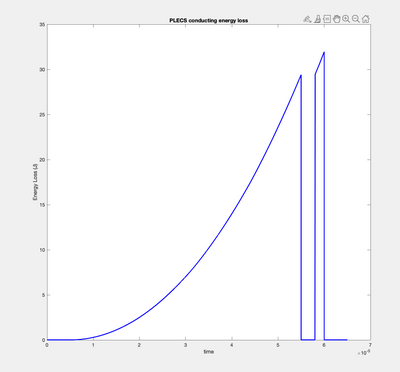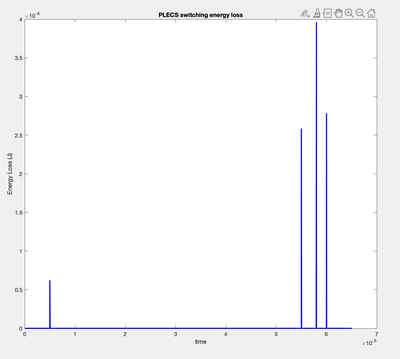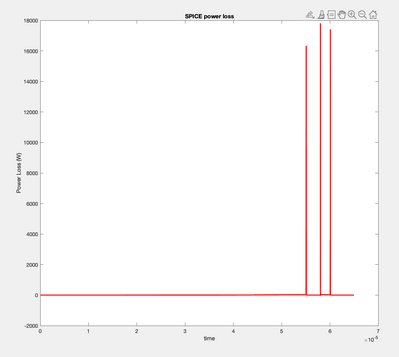2. I have changed the Vgs profile to be the same now, in SPICE the program allows for negative gate signal to ensure turn off but this is not used in PLECS. Both now use 0 V for turn off but it does not make a difference to the results. I have not added any parasitics to either circuit and they are as simple as they can be.

3. The Vds and Id graphs are very similar overall, the difference due to the ideal switching of the PLECS device can be seen when you zoom in though. Note: the test is done over 65 us.

Id: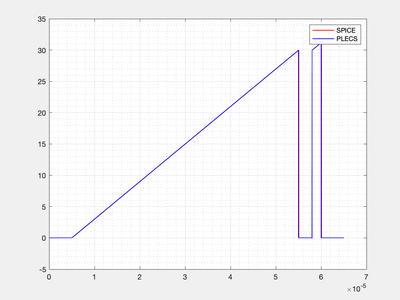Vds: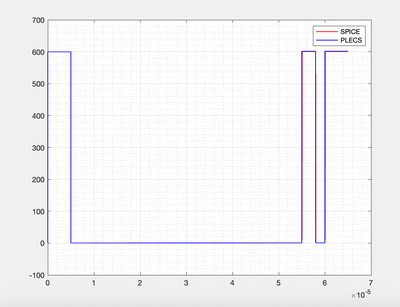Id zoomed: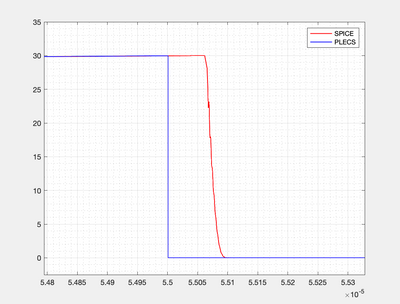4. I believe that in SPICE only the constant voltage input to Tc determines the temperature of the case. In PLECs I have set it up so the heatsink has a high enough thermal capacitance that it remains at the value of T of the constant T source. So both have input temperatures of 25C and the value of the resistance doesn't affect the junction temperature of the device. Let me know if there is another/better way of doing this.

Many thanks

HugoModeratorModerator

# Re: PLECS model vs SPICE model

Hello hugoc,

Thank you very much for your detailed explanation.
It helped us understand your problem.

Let us get rid of variables one by one, and see where the problem lies.

Firstly, the method of obtaining the energy loss (integration of Ploss).
You mentioned that the result by using the Matlab functions does not match with the results from PLECS.

LTSPICE has an inbuilt integration function.
You can use a behavioral voltage source as in the image below: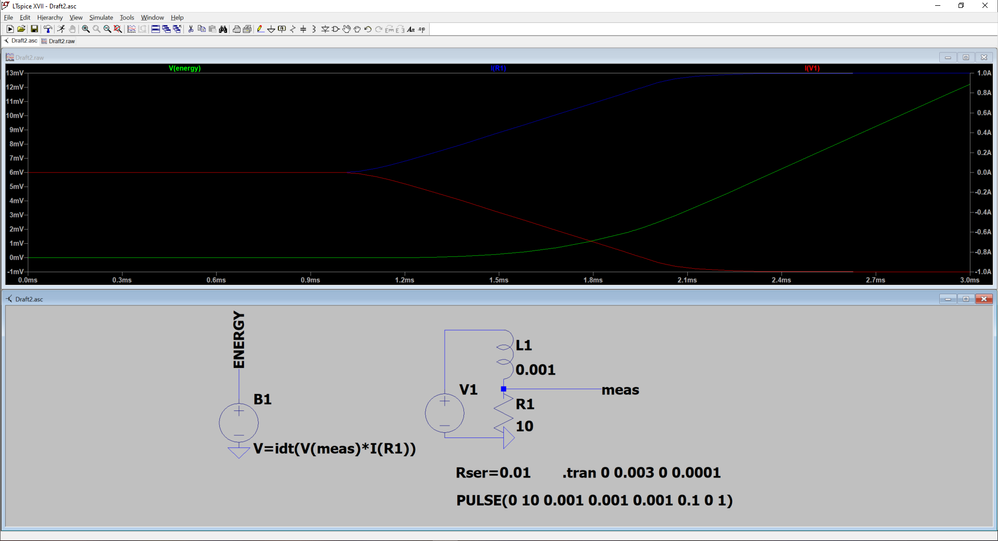As you can see, I set up a circuit with a transient state current.
The behavioral voltage source's expression includes the voltage sensed at point MEAS, as well as the current across R1.
This way we can obtain the power loss of R1.
The idt() expression gives us the integral of the power loss.
Therefore, by probing the output of the voltage source, we can plot the total energy loss.

Let us know if with this method the energy loss result is different.

Bets regards,
PabloLevel 1Level 1

# Re: PLECS model vs SPICE model

Hello Pablo,

Thankyou for this advice- it is a much easier way of doing the energy loss than I was doing previously! It gives me the same results as before though.

Could you suggest a method for turning the PLECS energy plots into something to compare the SPICE energy plot to? The PLECS conduction losses go up to 30 J whereas the switching only reaches 4e-4 J so simply adding them together gives basically the same plot as only conduction lossesModeratorModerator

# Re: PLECS model vs SPICE model

Hello Hugoc,

There is something wrong with the PLECS conduction losses.
These cannot be as high as 30J.

Just by simply integrating your Id waveform for the first pulse + second pulse:

Energy = Rdson*(Idpeak*Idpeak1*ΔT1/2 + Idpeak*Idpeak1*ΔT2 + (Idpeak2-Idpeak1)*(Idpeak2-Idpeak1)*ΔT2/2)
Energy = 0.03*(30*30*5e-5/2 + 30*30*2e-6 + (31-30)*(31-30)*2e-6/2)
Energy = 7.3e-4 J.

Could you check the settings of the PLECS block?

Best regards,
PabloLevel 1Level 1

# Re: PLECS model vs SPICE model

I agree there is a problem with these values and your calculations look much more correct. In terms of the settings, I have shown below the look up table within the Infineon model under conduction losses- there seems to be not much I can change relating to conduction loss. What this really shows is the change in rdson I believe without values for energy loss displayed.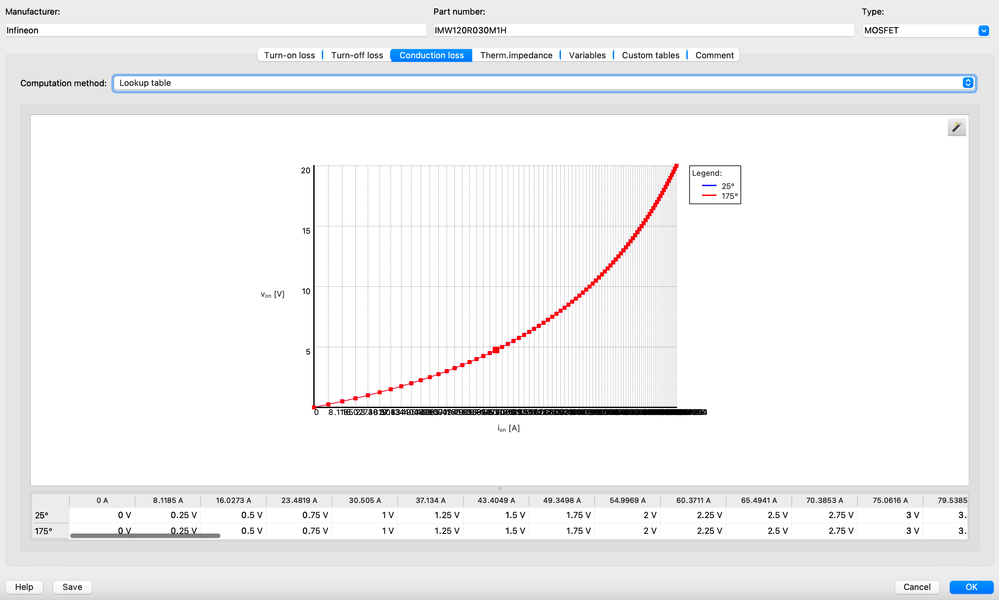Perhaps the outputs from the MOSFET model for the conduction losses are found through using  P=I^2 * R as this would give P = 30^2 * 0.03 = ~27  for the maximum point which is what can be seen in the output plots. What I have done that seems to work is use the conduction losses as power losses so integrating them and combining with the switching losses cumulatively gives the waveform in blue below (SPICE is in red). This is similar to the SPICE model ! However as you can see there are still differences as I found at the very start: this shows it may be due to the differences in how switching losses are calculated- especially at low currents (see first at 0.5e-5 s PLECS has large loss but SPICE is almost negligible). I don't know if this is just a difference between the software or the Infineon models as I think the test circuits are identical at this point.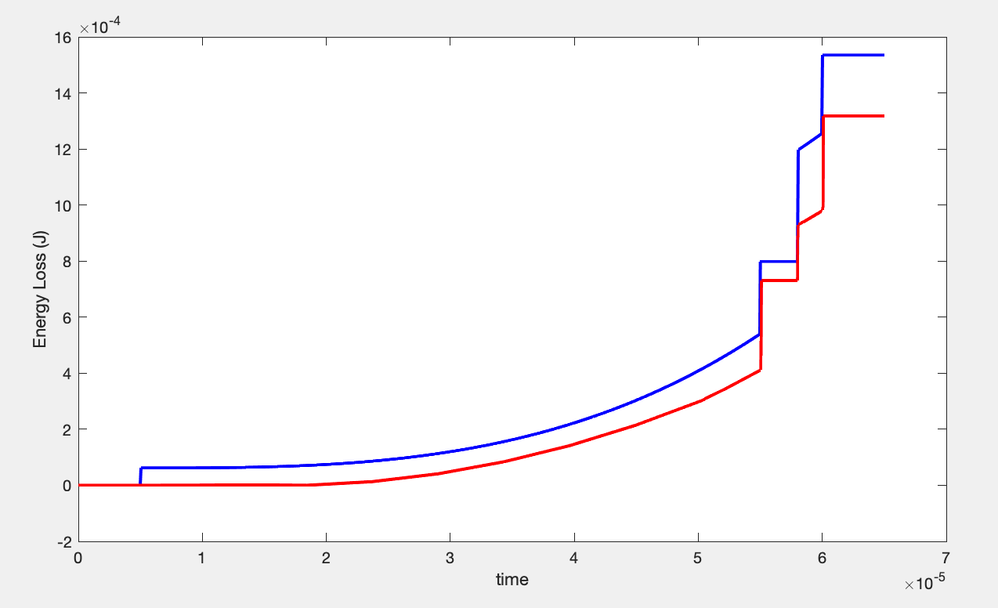ModeratorModerator

# Re: PLECS model vs SPICE model

Hello Hugoc,

We can conclude that the output of the PLECS simulation is power in Watts, and not energy in Joules.
I am glad you could get energy levels close to the SPICE simulation.

I understand that there is still a difference in the resulting energies.
As with time the error of the energy calculations will get bigger and bigger, I suggest you compare the instantaneous power instead.

Let me know about these three points:
1) Where did you obtain the simulation model for SPICE? On our product page, the simulation files provided are for the IMBGxxxxx series.
I assume you are using the IMBGxxxxx model. This is almost identical to the IMW part, but there are still differences in the switching losses.

2) When simulating and integrating the power from the PLECS output, what step size are you using? This can make a big impact on the resulting calculated energy.
Ideally, we would like to have a short a step as possible. Again, this problem will be lessened if just using power is the variable to compare.

3) Your SPICE simulation waveforms are very ideal. When simulating in SPICE on my side, there are some natural fluctuations due to the non-ideal nature of the components.
For example, the first turn-on should have some current peak.

4) This is more of a comment, but I went over the .xml file information.
Both turn-on and turn-off have a minimum energy loss assigned.
Therefore, even if the current through the device is 0A, there will be a significant energy loss recorded.
This is why we see a difference between SPICE and PLECS at the first turn-on.

Best regards,

Pablo